# Heat Transfer Networks¶

This section lists the heat networks generated by OpenModelica. The topologies are not differentiated, since OpenModelica can only create thermal networks to a limited extent. The individual pipes are mainly divided into 5 sections (sections = 5).

## Delta¶

Triangular network.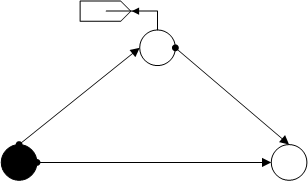`heat_transfer_delta`()
Returns

net - OpenModelica network converted to a pandapipes network

Return type

pandapipesNet

Example
```>>> pandapipes.networks.simple_water_networks.heat_transfer_delta()
```

## Delta with 2 Sinks¶

Triangular network with two sinks.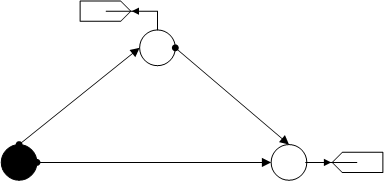`heat_transfer_delta_2sinks`()
Returns

net - OpenModelica network converted to a pandapipes network

Return type

pandapipesNet

Example
```>>> pandapipes.networks.simple_water_networks.heat_transfer_delta_2sinks()
```

## Heights¶

Cross-shaped net with height differences between the individual nodes. (sections = 7, for each pipe)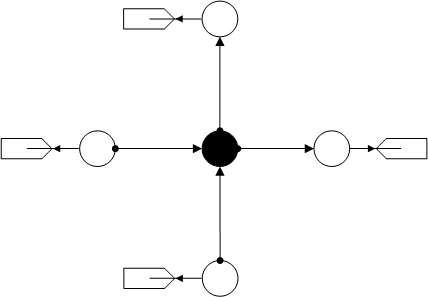`heat_transfer_heights`()
Returns

net - OpenModelica network converted to a pandapipes network

Return type

pandapipesNet

Example
```>>> pandapipes.networks.simple_water_networks.heat_transfer_heights()
```

## One Pipe¶

One pipe with a sink.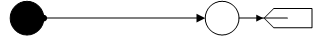`heat_transfer_one_pipe`()
Returns

net - OpenModelica network converted to a pandapipes network

Return type

pandapipesNet

Example
```>>> pandapipes.networks.simple_water_networks.heat_transfer_one_pipe()
```

## One Source¶

Strand net with one source and one sink.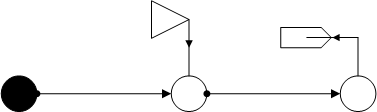`heat_transfer_one_source`()
Returns

net - OpenModelica network converted to a pandapipes network

Return type

pandapipesNet

Example
```>>> pandapipes.networks.simple_water_networks.heat_transfer_one_source()
```

## Section Variation¶

Mesh network with different values for “sections” of the four pipes (4, 7, 10, 16).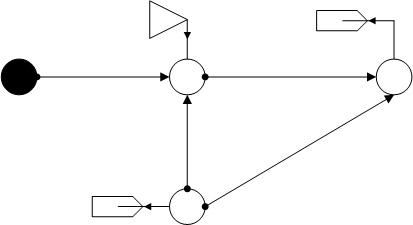`heat_transfer_section_variation`()
Returns

net - OpenModelica network converted to a pandapipes network

Return type

pandapipesNet

Example
```>>> pandapipes.networks.simple_water_networks.heat_transfer_section_variation()
```

## T-Cross¶

T-cross with two sinks.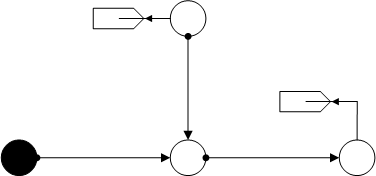`heat_transfer_t_cross`()
Returns

net - OpenModelica network converted to a pandapipes network

Return type

pandapipesNet

Example
```>>> pandapipes.networks.simple_water_networks.heat_transfer_t_cross()
```

## Two Pipes¶

Strand net with two sinks.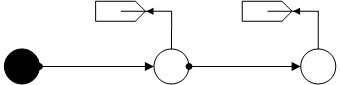`heat_transfer_two_pipes`()
Returns

net - OpenModelica network converted to a pandapipes network

Return type

pandapipesNet

Example
```>>> pandapipes.networks.simple_water_networks.heat_transfer_two_pipes()
```